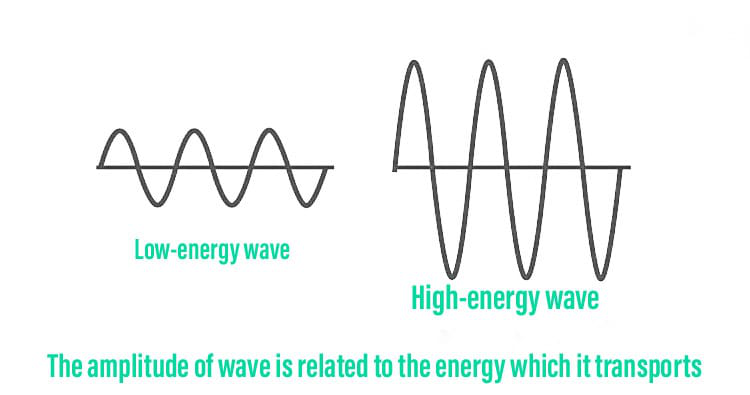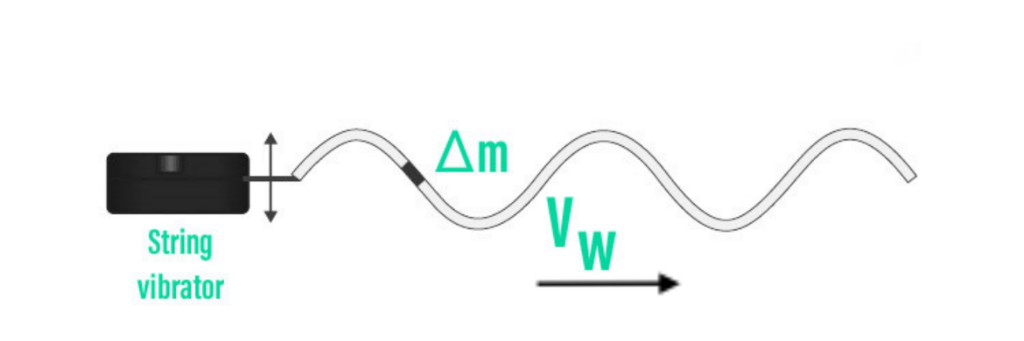GFG App
Open AppBrowser
Continue

Every wave carries energy. The energy of some waves, such as earthquake energy, maybe directly seen. Similarly, wave energy manifests itself in the churning of the coastline by sea waves and the pulverisation of nerve cells caused by strong noises. This article will look at the quantitative representation of energy in waves. In addition, we will learn what gives waves their energy and how amplitude and frequency connect to wave energy.

Wave motion is defined as the transmission of energy and momentum from one medium point to another without the actual transport of matter between the two sites. There are three distinct ways to classify wave motion:

• The propagation medium,
• The dimensions in which energy is propagated by a wave
• The transfer of energy

The conveyance and collection of energy by ocean surface waves are known as wave energy (or wave power). The captured energy is subsequently used for a variety of tasks, including electricity generation, water desalination, and water pumping. Wave energy is a sort of renewable energy that is projected to be the world’s largest ocean energy resource.

### What is the Wave Motion?

Wave motion is defined as the transmission of energy and momentum from one point in a medium to another without the actual transfer of substance between the sites.

Waves cause disruption as a function of time, and this function is referred to as the wave function. In the case of a string, it could be particle displacement, and in the case of sound waves, it could be a change in pressure or density.

Characteristics of Wave Motion

• A particle’s velocity is greatest at its mean location and zeroes at its extreme position.
• The velocity at which waves travel is not the same as the velocity at which particles vibrate.
• Disturbances propagate in the medium as a result of repetitive vibrations of the particles about their mean locations.
• Because each particle gets disturbed a little later than the one before it, there is a phase difference between the particles of the medium.
• Energy and momentum are moved from one location to another without any real transfer of the medium’s particles.

### Energy of wave

A wave’s energy is determined by two factors. The first is amplitude, which is the distance between a wave’s rest point and its top or bottom. Waves with a large amplitude contain more energy. The other factor is frequency, which is defined as the number of waves passing through each second.

In general, the amplitude squared and the angular frequency squared are proportional to the energy and power of a mechanical wave (and therefore the frequency squared). Mathematically,

I = P 4 π r2

The amplitude and frequency of a wave are connected to the amount of energy it contains. Earthquakes with enormous amplitudes cause massive ground displacements. Loud sounds have larger amplitude source vibrations and higher pressure amplitudes than mild sounds. The coastline is churned up more by large ocean breakers than by minor ones.

If two mechanical waves have comparable amplitudes but one has a frequency that is twice that of the other, the higher-frequency wave will transmit energy four times faster than the lower-frequency wave. It’s worth noting that, unlike mechanical waves, where the rate of energy transfer is proportional to both the square of the amplitude and the square of the frequency, electromagnetic waves have a rate of energy transfer that is proportional to the square of the amplitude but not to the frequency.

The following are some of the benefits of wave energy:

• Because waves come from far out at sea and can travel great distances without losing much energy.
• The power they generate is far more consistent and predictable from day to day and season to season.
• The kinetic energy of waves is nearly 1000 times that of wind.

### Relation between the Energy of a wave and Amplitude

The amplitude of a wave determines how much energy it can carry. A high energy wave has a large amplitude. In the same way, a low energy wave has a small amplitude. The amplitude and frequency of a wave are connected to the amount of energy it contains. The higher the seagull is carried by the wave and the greater the shift in potential energy, the larger the amplitude. Both the amplitude and frequency affect the wave’s energy.### Formula for Energy of a Wave

Take a look at the sinusoidal wave in the diagram. The amplitude and frequency of a wave determine its energy. Energy comes in two forms: kinetic and potential.Consider a string mass element with a mass of m. Because the string’s linear density is constant, each mass element has the same mass.

Δ m = μΔx

The sum of the wave’s kinetic and potential energy is its total mechanical energy. The kinetic energy is expressed as,

K = 1/4(μA2ω2λ),

where A is the wave’s amplitude (in meters), ω is the wave oscillator’s angular frequency (in hertz), and λ is the wavelength (in meters).

Thus, the total energy is given by,

Utotal = Upotential + Ukinetic

= 1/4 (μA2ω2λ) + 1/4 (μA2ω2λ)

= 1/2 (μA2ω2λ)

where A is the wave’s amplitude (in meters), is the wave oscillator’s angular frequency (in hertz), and is the wavelength (in meters).

### Sample Problems

Problem 1: What are mechanical waves?

Solution:

Mechanical waves are those that are generated by the vibration of material particles and must propagate across a medium. For example, sound waves, a vibrating string, and so on. Mechanical waves are caused by a disturbance in the medium, which propagates through the media without moving the medium’s material particles. In other terms, mechanical waves refers to waves that require a medium to travel or propagate.

Problem 2: A string with a mass of 2.50 kg is under 200 N of stress. The stretched string is 20.0 meters long. How long does it take for the transverse jerk to reach the opposite end of the string if it is struck at one end?

Solution:

Given that,

M = 2.50 kg is the mass of the string.

T = 200 N tension in the string

l = 20.0 m is the length of the string.

Mass per unit length, μ=M/l=2.50/20=0.125Kgm −1

The velocity (v) of the transverse wave in the string is given by the relation:

v=   √T/μ

= √200/0.125

=  √1600=40 m/s

∴ Time taken by the disturbance to reach the other end,

t=l/v=20/40=0.5s

Problem 3: A stone thrown from the top of a 300-meter-high tower splashes into the water of a pond near the tower’s base. Given that the speed of sound in air is 340 m s1, when is the splash heard at the top? (g = 9.8 m s2) (g = 9.8 m s2) (g = 9.8 m s)

Solution:

The tower’s height is 500 meters.

340 m/s is the sound velocity.

Gravitational acceleration, g = 10 m/s2

The stone’s starting velocity is u = 0. (since the stone is initially at rest)

t1 is the time it took for the stone to fall to the tower’s base.

According to the second equation of motion, the sound takes t2= 500/340 = 1.47 s to travel from the base of the tower to the top.

S = ut1 + 1/2gt12

500 = 0 x t1 + 1/2 x 10 x t12

t12 = 100

t1 = 10s

Therefore, the

The time it takes for the sound to travel from the base of the tower to the top is now t2= 500/340 = 1.47 s.

As a result, after some time, the splash can be heard from the top.

Where t1 + t2 = 10 + 1.47 = 11.47 seconds.

Problem 4: Give a brief definition of wavelength.

Solution:

A wavelength is the distance between two consecutive crests or troughs in a transverse wave, or two successive compressions or rarefactions in a longitudinal wave. A wavelength is the distance between any two successive particles of a medium in the same vibrating phase.

Problem 5: (i) Do all the points on a string oscillate at the same (a) frequency, (b) phase, and (c) amplitude for a wave on a string? Explain your responses.

(ii) What is the amplitude of a point 0.375 m from one end of the line?

Solution:

We know that, l = 1.5 m and l = 3 m for the wave on the string specified in questions. As a result, it is evident that = /2 is only conceivable for a string clamped at both ends if both ends act as nodes and there is only one antinode in between, i.e. the entire string vibrates in one segment.

(a) Yes, except for nodes, all ring particles vibrate at the same frequency of 60 Hz.

(b) Since all string particles are in the same segment, they are all in the same phase.

(c) The amplitude of each particle differs. Amplitude = 2A = 0.06 m at the antinode. It progressively descends.(c) The amplitude of each particle differs. Amplitude = 2A = 0.06 m at the antinode. It progressively descends.

Problem 6: What are the seven different types of waves?

Solution:

From the largest wavelength to the smallest, the electromagnetic spectrum contains radio waves, microwaves, infrared, optical, ultraviolet, X-rays, and gamma-rays

Problem 7: How is energy of wave is related to amplitude?

Solution:

The square of a wave’s amplitude is related to the quantity of energy it carries. As a result, whatever amplitude change takes place has an effect on the energy. When the amplitude is twice, this amounts to a quadrupling of energy.

My Personal Notes arrow_drop_up